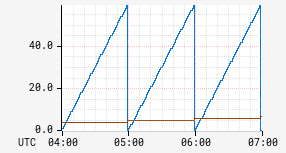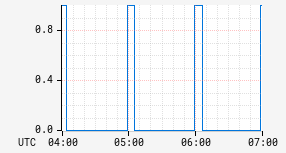# le

Less than or equal operator. There are two variants of the `:le` operator.

## Choosing¶

Input Stack:
 v: String k: String
Output Stack:
 (k <= v): Query

This first variant is used for choosing the set of time series to operate on. It selects time series that have a value for a key that is less than or equal to a specified value. For example, consider the following query:

```name,ssCpuSystem,:le
```

When matching against the sample data in the table below, the highlighted time series would be included in the result set:

Namenf.appnf.node
ssCpuUser nccp i-0abc
ssCpuSystem nccp i-0abc
numRequests nccp i-0abc
ssCpuUser api i-0456

## Math¶

Input Stack:
 ts2: TimeSeriesExpr ts1: TimeSeriesExpr
Output Stack:
 (ts1 <= ts2): TimeSeriesExpr

Compute a new time series where each interval has the value `(a <= b)` where `a` and `b` are the corresponding intervals in the input time series. For example:

Time a b a <= b
00:01 0.0 0.0 1.0
00:01 0.0 1.0 1.0
00:02 1.0 0.0 0.0
00:03 1.0 1.0 1.0
00:04 0.5 1.7 1.0

The result will be a signal time series that will be `1.0` for intervals where the condition is true and `0.0` for intervals where it is false.

Example:

 Before After```minuteOfHour,:time, hourOfDay,:time ``` ```minuteOfHour,:time, hourOfDay,:time, :le ```